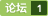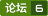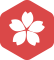### python中使用pylab画图，代码无报错，但没有图像显示，怎么办 [问题点数：40分]pylab.show()没有显示图形图像（python的matplotlib画图包）
no display name and no \$DISPLAY environment variable ============================ @Neil's answer is
python用plot画图困扰了一个晚上，实在搞不懂，求大佬们救救孩子!
row_n = 0 a = text = open('D://PythonCodes//PM2.5Prediction//ans.csv', 'r', encoding = 'big5') row =
pycharm执行图形程序不出现图形界面
-
tensorflow 中的python错误：没有模块命名为pylab
1.tensorflow 中的<em>python</em>错误：<em>没有</em>模块命名为<em>pylab</em> import <em>pylab</em> <em>报错</em><em>没有</em><em>pylab</em>这个模块，解决办法： 需要安装numpy，scipy和matplotlib来获取<em>pylab</em>。在ubuntu中，您可以<em>使用</em>此命令安装它们： Python版本是<em>python</em> 2.7<em>使用</em>如下命令： sudo apt-get install <em>python</em>-numpy <em>python</em>-...
python数据分析之（7）简单绘图pylab

Python 使用 pylab 库实现画线功能的方法详解

Pycharm中使用matplotlib绘图不能显示
<em>使用</em>Pycharm中<em>使用</em>matplotlib绘图时，要想显示绘图需要加上matplotlib.pyplot.show()方法。 以下<em>代码</em>在Pycharm中运行不会显示绘图：import matplotlib.pyplot as plt import numpy as np plt.plot(np.arange(10))以下<em>代码</em>在Pycharm中运行现实绘图：import matplotlib.py

import plotly import plotly.plotly as py from plotly.graph_objs import * plotly.tools.set_credentials_file(username='JaneGinkgo', api_key='IDOV3Q7jOtABYvw7mhin') trace0 = Scatter( x=[1, 2, 3, 4

python使用plot画图，图不显示

【Python开发】matplotlib绘图不显示问题解决plt.show()

Python 中，matplotlib绘图无法显示中文的问题
Python 中，matplotlib绘图无法显示中文的问题
Matplotlib 远程画图报错

python pylab画图工具
<em>pylab</em> 是 matplotlib 面向对象绘图库的一个接口，它的语法和 Matlab 十分相近，主要的绘图命令和 Matlab 对应的命令有相似的参数 form <em>pylab</em> import * # 普通<em>画图</em> X = np.linspace(-np.pi, np.pi, 256,endpoint=True) C,S = np.cos(X), np.sin(X) plot(X,C) plot...
Python绘图问题：Matplotlib中%matplotlib inline是什么、如何使用
%matplotlib inline 是一个魔法函数（Magic Functions）。官方给出的定义是：IPython有一组预先定义好的所谓的魔法函数（Magic Functions），你可以通过命令行的语法形式来访问它们。可见“%matplotlib inline”就是模仿命令行来访问magic函数的在IPython中独有的形式。magic函数分两种：一种是面向行的，另一种是面向单元型的。 行m

RFCN python demo.py 文件 运行后 没有检测结果图片显示 ?

python2.7.5 画图问题，图形不显示
-
pandas中强大的绘制图表功能——DataFrame.hist()，以及统计分析函数——DataFrame.describe()
pandas中具有很多很强大的图表绘制功能，今天介绍一个简单好用的绘制数据框DataFrame中某一列数据分布直方图的函数——DataFrame.hist()，顺便介绍一个统计分析函数DataFrame.describe()。 下面通过<em>代码</em>进行展示效果，示例<em>代码</em>中的csv文件是谷歌提供的一个包含加利福尼亚州住房数据的文件。 import pandas as pd california_ho...
python之matplotlib中plt.show()不显示图的解决办法

PyLab Python模块,属于Matplotlib数学库。PyLab结合数值与图形绘制模块模块numpy pyplot。PyLab交互式Python解释器的设计思想,因此它的许多功能是很经典的（属于小而巧那种）。这使得它非常有效和方便的数学工具。如果你想安装PyLab,您还必须安装Matplotlib。这个过程相当简单,采用标准的安装过程； windows下的安装：pip install -U
matplotlib的常用的两种方式以及pylab
<em>pylab</em>不推荐<em>使用</em>的原因：<em>pylab</em>更接近于MATLAB，这是毋庸置疑的，但是<em>使用</em><em>pylab</em>会逐渐背离matplotlib的学习，这与初衷想反，当然，还有其他的原因，<em>没有</em>研究。 三种方式的实现<em>代码</em> pyplot的方式 #!/usr/bin/<em>python</em> #coding: utf-8 import numpy as np import matplotlib.pyplot a
pycharm无法显示matplotlib绘图问题

ubuntu16.04下python3pylab模块安装不成功，无tkinter模块

python可视化——matplotlib画图颜色控制

Pycharm Matplotlib 图像不显示问题

MAC 使用pycharm出现ImportError: No module named numpy 解决方法
Mac OS X 10.11.5 问题描述： 我用的是anaconda安装<em>python</em>3.6 已经在终端 pip install numpy 但是在pycharm运行程序出现错误：ImportError: No module named numpy  解决方法： 首先在终端输入 \$which <em>python</em>3.6 获得安装路径后。 然后在pycharm的preference
Python与PyCharm的中文显示问题

python之matplotlib中plt.show()不显示

python中运行代码没有报错但是也没有输出而且还有exit code 0的结束标志
f=open(&quot;passwd.txt&quot;,'r') print (f.read(4)) f.close() 这是想要执行的<em>代码</em> passwd.txt中的内容 ntp:x:38:38::/etc/ntp:/sbin/nologin apache:x:48:48:Apache:/var/www:/sbin/nologin saslauth:x:498:76:Saslauthd user:/var/em...
matplotlib绘图实例：pyplot、pylab模块及作图参数
http://blog.csdn.net/pipisorry/article/details/40005163 Matplotlib.pyplot绘图实例 {<em>使用</em>pyplot模块} matplotlib绘制直线、条形/矩形区域 import numpy as np import matplotlib.pyplot as plt t = np.arange(-1, 2, .01) s = ...
Matplotlib绘图：pylab模块中部分函数参数说明

hello world代码明明正确，运行也没有报错，pycharm为什么没有输出正确的结果

Anaconda Spyder下Ipython console画图出不来图
Anaconda Spyder中写好<em>画图</em>的<em>代码</em>，有时候并不会直接在I<em>python</em> console中出来图训练的过程，我们需要在I<em>python</em> console中输入以下命令才可以：（1）直接在窗口输出图片结果：在I<em>python</em> console中输入命令:In:matplotlib inline（2）在图片小窗口输出动图图片：在I<em>python</em> console中输入命令:In:matplot...
Seaborn简单画图(四) -- 设置图形显示效果
import numpy as np import pandas as pd import matplotlib.pyplot as plt %matplotlib inline x = np.linspace(0,14,100) y1 = np.sin(x) y2 = np.sin(x+2)*1.25 def sinplot(): plt.plot(x,y1) plt.pl...
1-python图像处理之PIL，pylab
<em>python</em>计算视觉学习基础，PIL和<em>pylab</em>类库的简单<em>使用</em>，一. 读图与显示1.<em>使用</em><em>pylab</em>中的 imread() 读图img = imread('图片路径')或者<em>使用</em>PIL中Image的open()方法img = Image.open('图片路径')2.显示<em>使用</em><em>pylab</em>中的 imshow()imshow(img)或者 <em>使用</em>Image中的类（和上面不一样）img.show()测试用例
python matplotlib 图像显示问题

seaborn在pycharm中绘图不出图原因
import numpy as npimport seaborn as sns import matplotlib.pyplot as plt np.random.seed(sum(map(ord, 'axis_grids'))) tips = sns.load_dataset('tips') tips.head() # 将FacetGrid实例化出来 g = sns.FacetGrid(ti
python pylab 画图 图例 多折线图
import <em>pylab</em> as pl   def drawFigure(list,nameslist): linestyle=['cx--','mo:','kp-.','bs--','p*:'] #红，绿，黄，蓝，粉,每个折线给不同的颜色 linenum=len(list) ...
python matplotlib画图，无法显示图像

Jupyter 不执行代码，显示In[*]

python matplotlib 画图show()不显示图

python用plotly绘制折线图为什么跑下来没有
-
Mac 使用python3的matplot画图不显示问题

python matplotlib中文显示乱码解决

Python运行正常但是没有结果

python基本图像操作
Python基本图像操作<em>使用</em><em>python</em>进行基本的图像操作与处理前言：与早期计算机视觉领域多数程序都是由 C/C++ 写就的情形不同。随着计算机硬件速度越来越快,研究者在考虑选择实现算法语言的时候会更多地考虑编写<em>代码</em>的效率和易用性,而不是像早年那样把算法的执行效率放在首位。这直接导致近年来越来越多的研究者选择 Python 来实现算法。今天在计算机视觉领域,越来越多的研究者<em>使用</em> Python 开展研
【工具使用系列】Python FAQs
Python FAQs
python3使用matplotlib画图支持中文

python程序运行卡住的问题

Python导入matplotlib找不到指定的模块

linux 服务器没有GUI时如何使用matplotlib绘图

Linux服务器没有GUI的情况下使用matplotlib绘图
import matplotlib as mpl mpl.use('Agg') #而且必须添加在import matplotlib.pyplot之前，否则无效 #最后在plt.draw后面加上plt.savefig("/home/yourname/picFaster.jpg") #就可以把绘制的图存为jpg，down到本地查看就行了

Python中文画图乱码的解决方法
&nbsp;&nbsp;&nbsp;&nbsp;&nbsp;&nbsp;&nbsp;&nbsp;在Python<em>画图</em>时，我们一般希望在图中添加中文标签或者变量名称，这样可以增强我们图形的可读性，可是在Python中有时会出现了乱码。 &nbsp;&nbsp;&nbsp;&nbsp;&nbsp;&nbsp;&nbsp;&nbsp;下面举出一个例子说明如何解决中文乱码的问题。 import pandas...
python2.7之matplotlib中plt.show()不显示图的解决办法

pylab模式”打开如何解决">利用python进行数据分析——p26,"一定要以pylab模式”打开如何解决

Pandas绘图
Pandas绘图Pandas的绘图方法封装了Matplotlib的pyplot方法，可以提供简单的绘图功能,对于DataFrame来说，.plot是一种将所有列及其标签进行绘制的简便方法不常用，实际应用中，一般仍<em>使用</em>Matplotlib绘图Jupyter notebook中如不显示Pandas绘制图像，解决方法: 载入import Matplotlib.pyplot as plt，Pandas绘图代
Linux下Octave使用画图报错的解决方法

<em>使用</em>pycharm的matplotlib函数时出现如下<em>报错</em>：解决办法：1、查看并确认<em>python</em>环境是否成功安装matplotlib本人<em>使用</em>anaconda3.5.1已经安装好2、打开控制面板 - &amp;gt;系统设置 - &amp;gt;高级系统设置 - &amp;gt;环境变量-&amp;gt;系统变量 - &amp;gt;新建。设置变量名称：QT_PLUGIN_PATH，变量目录：...\Anaconda2 \ Library...
python学习笔记（十）错误处理、Matplotlib绘图示例

python绘图时ion和ioff的用法
# -*- coding: utf-8 -*- import numpy as np import matplotlib.pyplot as plt import time x = np.linspace(-1, 1, 100) y = np.sin(x*np.pi) fig = plt.figure() ax = fig.add_subplot(1, 1, 1) plt.ion() fo...

Linux终端没有GUI，如何使用matplotlib绘图

Python使用matplotlib进行可视化时精确控制图例位置

Python杂谈 | (2)Python3中使用Matplotlib绘图时的中文乱码问题

python中 matplotlib.pyplot 库中 imshow 方法——画灰度图

python 画图--简单开始及折线图
<em>python</em> <em>画图</em> 一、环境准备            linux ubuntu 下需安装下面三个包：                   Numpy, Scipy,Matplotlib          分别输入下面的<em>代码</em>进行安装： pip install numpy pip install scipy sudo apt-get install <em>python</em>-matpl
python画图的scatter，以及各种属性设置详解，也可以用到plot中
http://blog.csdn.net/anneqiqi/article/details/64125186
pycharm的python包opencv（cv2）无代码提示问题的解决
pip install opencv-contrib-<em>python</em> 修改‪xxx\Anaconda3\Lib\site-packages\cv2\__init__.py为： import sys import os import importlib os.environ[&amp;amp;amp;amp;amp;quot;PATH&amp;amp;amp;amp;amp;quot;] += os.pathsep + os.path.dirname(os.path.realpath(__fi...

<em>使用</em>pygal<em>画图</em> 今天需要用<em>python</em>画一些数据比较的图，上网对比完<em>python</em>的图像库后决定选用pygal（考虑到 上手的难易度，和我对图形暂时<em>没有</em>复杂的需求） pygal文档 <em>python</em>安装 pip install pygal 基本<em>使用</em>方式 bar = pygal.Bar() bar.title = &quot;bar测试&quot; bar.x_lab...

<em>pylab</em>是<em>python</em>下挺不错的一个<em>画图</em>模块，<em>使用</em>也非常简单，记得Mit的计算机科学及编程导论有节课也是用到了这个工具，但这个工具安装不象用起来那么方便，小编就图文全程直播下吧 工具/原料 <em>python</em>2.7.10 win10 32位 方法/步骤 1 缺省状态下<em>python</em>安装
Python使用pylab库实现绘制直方图功能

Python手绘图案出现MemoryError错误、缺少numpy、PIL包的问题
MemoryError问题 出现MemoryError问题是图像质量高，而电脑配置跟不上，就会出现内存不够用的情况，出现MemoryError，解决的好办法就是你换个配置高的电脑试试（哈哈哈。。开玩笑）初学换个简单的图片就可以，直接截图保存一张，就可以了，或者把高质量图片用<em>画图</em>调像素、或者用ps等等软件都可以调。 缺少numpy、PIL 打开cmd输入命令就可以安装，如果不能直接安装请翻墙。...
PyCharm远程开发调试，解决mtplotlib不显示图像问题。

SAP中执行没有权限的事务

【Python开发】使用pyplot模块绘图

Python高级数据处理与可视化（六）---学科应用

python3.x版本的图像输出的结点中文显示问题

python中scatter（）函数用法，matplotlib画图
scatter函数用于绘制散点图。 scatter函数原型 matplotlib.pyplot.scatter(x,y,s=20,c='b',maker='o',cmpa=None,norm=None,vmin=None,vax=None,alpha=None,linewidths=None,verts=None,hole=None) 函数中各个参数介绍 x,y是相同长度的数组。 s
sublime解决使用快捷键Ctrl+B运行python程序只出现空白框但是却没有其他结果输出的问题

Ubuntu 16.04无界面使用matplotlib画图报错解决方案
Ubuntu 16.04命令行模式下运行含有matplotlib的<em>python</em>脚本<em>报错</em>:_tkinter.TclError: no display name and no \$DISPLAY environment variable解决方法:在 from matplotlib import pylot 之前，添加<em>代码</em>：如下：import matplotlib as plt plt.use('Agg'...
TinySeal3 cocos2d-x下载

readyAPI破解key下载